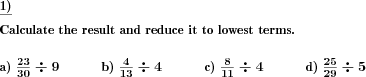Custom math worksheets at your fingertips# Details for problem "Divide a fraction by a whole number"

Quickname: 5220

Elementary School, Primary School, Junior High School, Middle School, High School.

## Summary

A fraction has to be divided by a whole number.

## Example## Description

A fraction has to be divided by a whole number. The number range for the numerators and denominators of the reduced fraction can be specified. After converting the result to like quantities, the numerator and denominators of the unreduced result may be up to the square of the range.

Improper fractions can be allowed, disallowed or enforced. In case they may occur, it can be chosen whether they will be output as improper fractions or mixed numbers.

The task may be formulated to ask for the result to be reduced to lowest terms.

The number of problems is selectable.

Download free printable worksheets for this math problem here. The worksheet contains the problems only, the solution sheet includes the answers. Just click on the respective link.

•Worksheet 1Solution sheet with answers
•Worksheet 2Solution sheet with answers
•Worksheet 3Solution sheet with answers

If you can not see the solution sheets for download, they may be filtered out by an ad blocker that you may have installed. If this is the case, please allow ads for this page and reload the page. The solution sheets will then reappear.

• Do these sample worksheets do not really fit?
• Do you need more math worksheets, with a different level of difficulty?
• Would you like to combine different problems on a worksheet and adjust them to your needs?
• As a teacher, you can put together your own worksheets using the automatically generated math problems provided.
With a free initial credit, you can start creating your own math worksheets in a few minutes.

You can try it for free! Register here, to create custom worksheets now!

## Customization options for this problem

Parameter
Possible values
Number of problems
1, 2, 3, 4, 5, 6, 7, 8, 9, 10
Number range
10, 20, 30, 40, 50, 100, 200, 500, 1000, 10000, 100000
Improper fractions
No, Yes, as a fraction, Yes, as a mixed number
Yes, No

## Similar problems

Remark
Description
A variant exists with the divisor being a fraction
Division of two fractions.Deutsche Version dieser Aufgabe
These informational pages with samples describe math problems that can be combined on custom math worksheets with solutions for home and K-12 school use.
Deutsche Seiten
×